# Two pumps

The first pump fills the tank in 24 hours second pump in another 40 hours. For how long will it take to fill the tank if it operates both pumps at the same time?

x =  0 h

### Step-by-step explanation:

Equation is non-linear.

## Calculation:

Equation is linear and have one solution:

8x-120=0

x=15

Our simple equation calculator calculates it.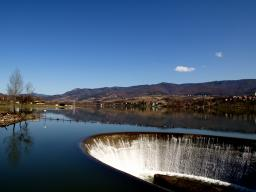Did you find an error or inaccuracy? Feel free to write us. Thank you!Tips to related online calculators
Looking for calculator of harmonic mean?
Looking for a statistical calculator?
Do you have a linear equation or system of equations and looking for its solution? Or do you have a quadratic equation?
Do you want to convert time units like minutes to seconds?

## Related math problems and questions:

• Pumps A and BPump A fill the tank for 12 minutes, pump B for 24 minutes. How long will take to fill the tank if he is only three minutes works A and then both pumps A and B?
• Three pumpsWe are filling the pool. The first pump would be filled in 12 hours, the second pump in 15 hours. If all three pumps were running at the same time, it would fill the pool for 4 hours. How long would the pool fill only with the third pump?
• Two pumps togetherThe first pump will fill the tank itself in 3 hours and the second one in 6 hours. How many hours will the tank be full if both pumps are worked at the same time?
• PumpingThe tank is filled with a larger pump in 12 hours and with scond pump in 15 hours. For how long does the tank fill when both pumps are switched on at the same time?
• Two pumpsPump A takes twice as long to fill a tank as B. Together they take 2 hours to fill the tank. How long does each one take to fill the tank by itself?
• Five pumps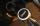Three same pumps fill the tank with 50400 liters of diesel in 7 hours. How many liters of diesel will it take in 4 hours if we add two more of the same pumps and pump them the same way? How much more (or less) will they get if we add 2 of the same pumps a
• Three pumps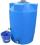The water tank is emptied with the first pump in 6 hours, with the second pump in 2 hours. In order to be emptied in 1 hour, all three pumps had to be started at once. How long will take tank be emptied by only the third pump?
• Three pumps together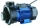One pump fills the tank in 1.5 hours, the second in 2 hours, and the third in 3 hours 20 minutes. How many minutes will the tank fill with three pumps if they work simultaneously?
• Tanker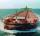An oil tanker can be emptied by the first pump in 4.9 hours. The second pump can empty a tanker in 8 hours. If the first pump started at 4:00 and the second 1.4 hour later, at what time will the tanker be empty?
• Harvesters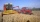The first harvester will harvest the grain from the field in 20 hours, the second, the more powerful in 10 hours. How long will it take to harvest this field by both harvesters if the second harvester has to be set up first and this takes 2 hours? The fir
• Inflow - outflow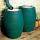The tank will fill by inflow for 143 minutes and empties by outflow for 150 minutes. How long take to fill tank if it is also opened the inflow and outflow?
• The dam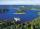The water reservoir is filled with first tributary for 25 hours second tributary for 35 hours. Some time were both inlets open, then a second tributary closed and the tank was filled in four hours. What time water flowed from both tributaries? (Expressed
• Pumps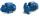Four identical pumps fill the tank in 40 hours. How many pumps would we have to use if we wanted to save 8 hours?
• Tributaries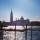The first tributary fill pool with water in 15 hours. The second tributary fill pool in 10 hours. For how many hours the pool is filled with both tributaries?
• Water reservoirThe water reservoir is filled with one pump for 4 days by the second one for 9 days. Outlet can drain tank in 12 days. How long take fill the reservoir if both pumps are running and not closed outlet channel.
• Machines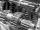In the workshop are three machines. The first is treated 250 parts for 4.7 hours, the second is treated 300 parts for 1.9 hours, and the third is treated 230 parts for 4.6 hours. How long will it take to make 3100 parts if worked all three machines at the
• Five pumpsThe water tank is filled with two pumps in 48 minutes. How long would it take to fill it with 5 same pumps?